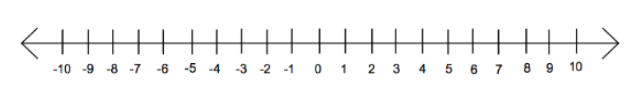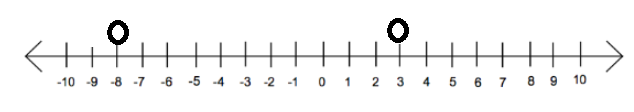# What is an Integer?

Integers are positive whole numbers and their opposites, including zero.

You might be wondering, what is an opposite?

The opposite of 2 is -2.

The opposite of 24 is -24.

The opposite of -13 is 13.

The opposite of 0... Well, it is just 0.

There is no opposite because zero is neither positive nor negative.

We can list the integers on a number line and then add arrows to show that integers continue on and on in both directions.Integers are helpful when modeling real life situations. Here are some examples:We can compare integers the same way that we compare positive whole numbers. Start by using a number line. The farther the number is to the right, the larger the value is.

Compare -9 and -2.Here, -2 is farther to the right. Therefore, -2 is greater than -9. -2 > -9.

Compare -8 and 3.

You might be tempted to say that -8 is larger than 3 because 8 is larger than 3. Let's first look on the number line.Here we can see that 3 is larger than -8. 3 > -8.

A positive number will always be greater than a negative.

Integers will become helpful when solving problems like those that include temperatures (above and below zero degrees), heights above and below sea level (sea level acts as 0), or even when we work with money (where less than 0 shows we owe someone money).